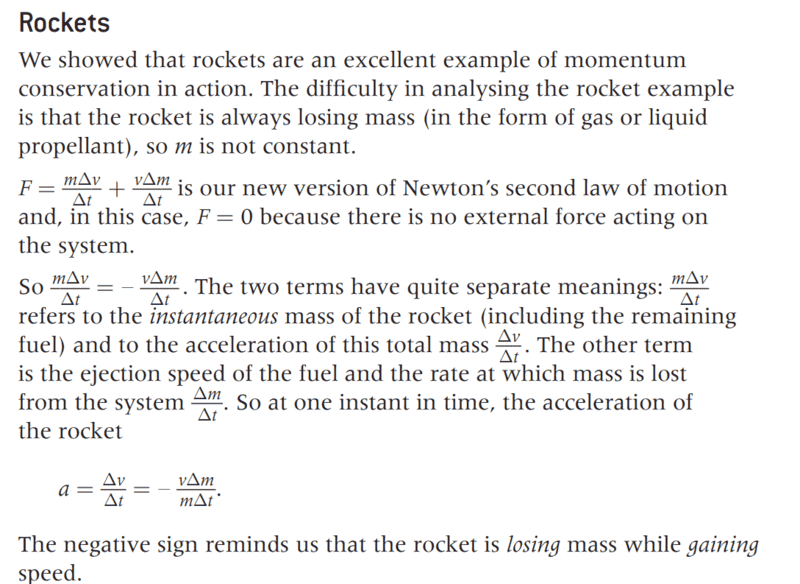# Newton's second law -- rockets

• B
Woopa
I am having difficulty understanding the information below. In the second line it states that F=0 as there is no external force on the system. However it later calculates acceleration of the rocket.

How can Force=0 if there is acceleration? (This is the first time I have encountered the product rule so this may be part of my misunderstanding)Gold Member
The keyword here is "system". By considering both the propellant (initial at rest inside the rocket) and the rocket itself to be part of the system and then say this system is isolated we are effectively saying that the net force between the mass inside and any mass outside the system is zero, leaving us to consider only forces between mass inside the system. This then means the momentum of the system is unchanged, or equivalently, if one part of the system, e.g. some of the propellant, accelerate one way then another part, e.g. the rocket plus its remaining propellant, has to accelerate in the opposite direction.

•topsquark, Ibix, PeroK and 1 other person
Homework Helper
Gold Member
2022 Award
Note that the "new form" of Newton's second law given here is not valid in general. There are several threads on here analysing this question.

In fact it's not really Newton's second law, but an equation that is only valid in this specific case.

PS the author has confusingly used the same letter ##v## for the velocity of the rocket and the velocity of the expellant. A simpler way to look at this is to use conservation of momentum:
$$m_r\Delta v_r + \Delta m_e v_e = 0$$Where we need the assumption that ##\Delta m_e## is small compared to ##m_r##. Otherwise, we would need to be more explicit that the mass of the rocket is changing from ##m_r + m_e## to ##m_r## after the expellent is fired out.

Last edited:
•Filip Larsen, vanhees71 and topsquark
2022 Award
•erobz, vanhees71, PeroK and 1 other person
Homework Helper
Gold Member
2022 Award
PS if we are being harsh, then the author has made two mistakes which cancel out and contrive to get the right equation:

1) Wrongly stating Newton's second law as ##F = m\frac{\Delta v}{\Delta t} + v\frac{\Delta m}{\Delta t}##.

2) Mistakingly using the change in velocity of the rocket as ##\Delta v## and the velocity of the expellant as ##v## in this equation.

•erobz, Ibix and vanhees71